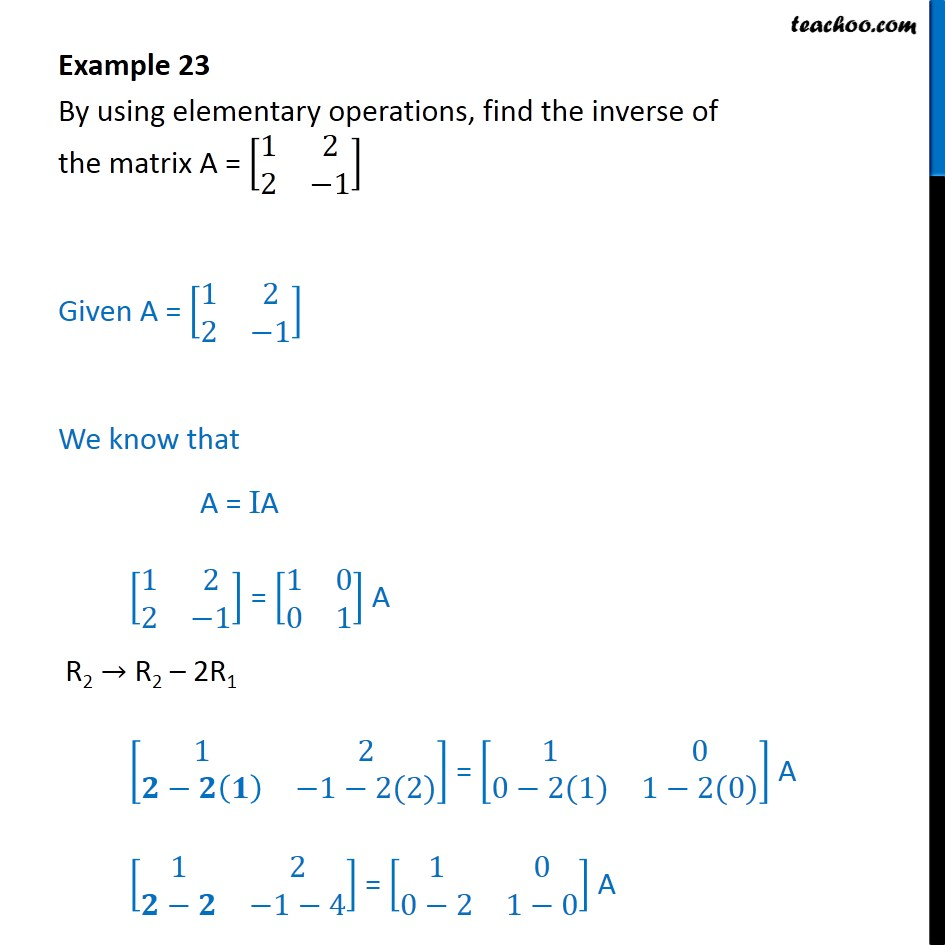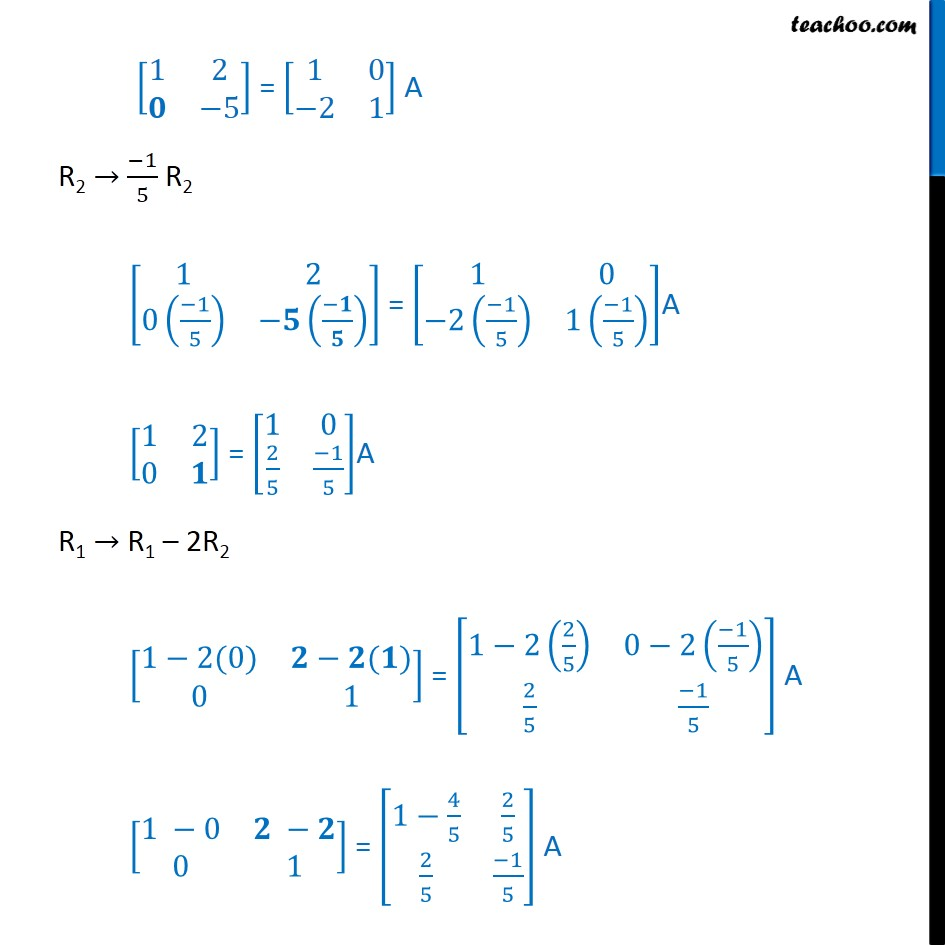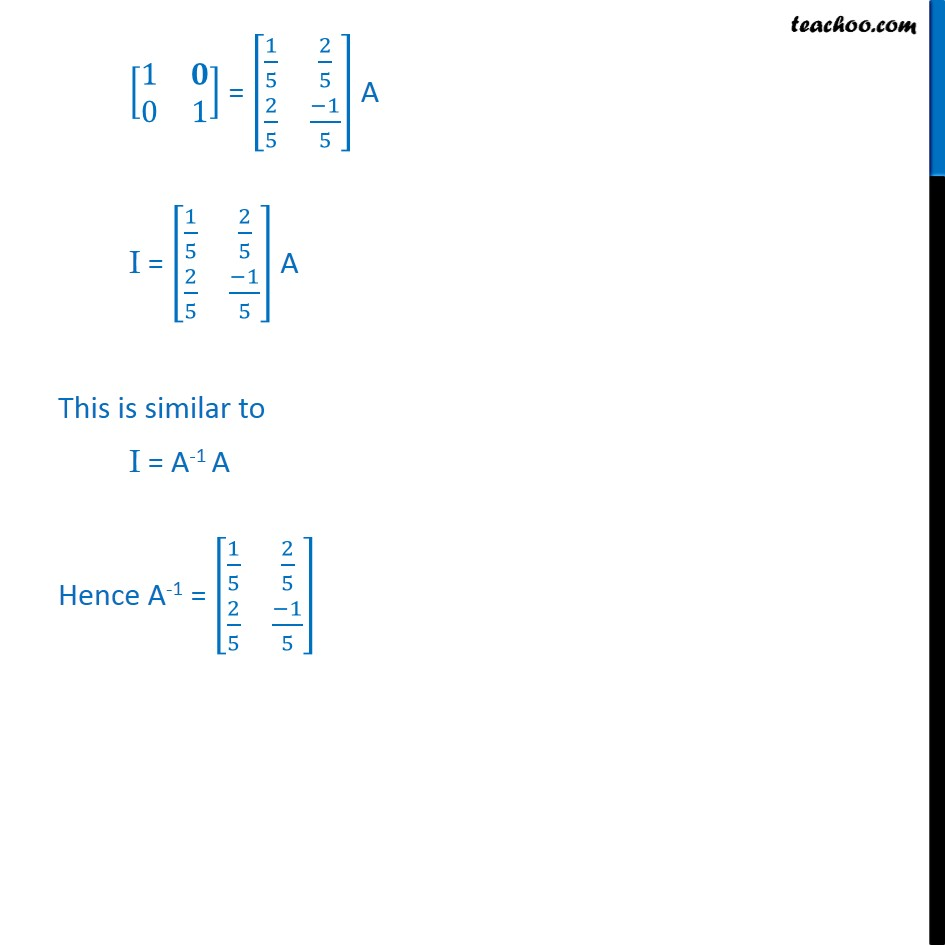Examples

Chapter 3 Class 12 Matrices
Serial order wiseLearn in your speed, with individual attention - Teachoo Maths 1-on-1 Class

### Transcript

Question 1 By using elementary operations, find the inverse of the matrix A = [■8(1&2@2&−1)] Given A = [■8(1&2@2&−1)] We know that A = IA [■8(1&2@2&−1)] = [■8(1&0@0&1)] A R2 → R2 – 2R1 [■8(1&2@𝟐−𝟐(𝟏)&−1−2(2))] = [■8(1&0@0−2(1)&1−2(0))] A [■8(1&2@𝟐−𝟐&−1−4)] = [■8(1&0@0−2&1−0)] A [■8(1&2@𝟎&−5)] = [■8(1&0@−2&1)] A R2 → (−1)/5 R2 [■8(1&2@0((−1)/5)&−𝟓((−𝟏)/𝟓) )] = [■8(1&0@−2((−1)/5)&1((−1)/5) )]A [■8(1&2@0&𝟏)] = [■8(1&0@2/5&(−1)/5)]A R1 → R1 – 2R2 [■8(1−2(0)&𝟐−𝟐(𝟏)@0&1)] = [■8(1−2(2/5)&0−2((−1)/5)@2/5&(−1)/5)] A [■8(1 −0&𝟐 −𝟐@0&1)] = [■8(1−4/5&2/5@2/5&(−1)/5)] A [■8(1&𝟎@0&1)] = [■8(1/5&2/5@2/5&(−1)/5)] A I = [■8(1/5&2/5@2/5&(−1)/5)] A This is similar to I = A-1 A Hence A-1 = [■8(1/5&2/5@2/5&(−1)/5)]